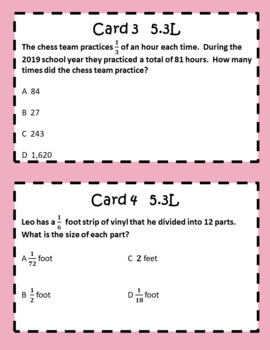# 5th Grade STAAR EOC TEK Aligned Task Cards 5.3 F,H,L AND 5.4 B,C,DSubject
Resource Type
File Type

PDF

(742 KB|N/A)
Product Rating
Standards
• Product Description
• StandardsNEW

TEK aligned task cards are the perfect resource to help engage your students and pass the 5th grade STAAR/EOC. No more searching for extra practice problems. These multiple-choice task cards look similar to the test problems and use much of the same wording and vocabulary. Spiral these cards throughout the year. Answers and recording sheet are included.

This resource includes:

20 STAAR aligned cards labeled by TEK

5.3L (5 cards)…Readiness (divide whole numbers by unit fractions and unit fractions by whole numbers)

5.4B (5 cards)…Readiness (represent and solve multi-step problems involving the four operations with whole numbers using equations with a letter standing for the unknown quantity)

5.4C (5 cards)…Readiness (generate a numerical pattern when given a rule in the form y = ax or y = x + a and graph)

5.4D (1 card)…Supporting (recognize the difference between additive and multiplicative numerical patterns given in a table or graph)

5.3F (2 cards)…Supporting (represent quotients of decimals to the hundredths, up to four-digit dividends and two-digit whole number divisors, using objects and pictorial models, including area models)

5.3H (2 cards)…Supporting (represent and solve addition and subtraction of fractions with unequal denominators referring to the same whole using objects and pictorial models and properties of operations)

Multiple Choice and TEK Aligned

Student Recording Sheet

Task Cards have many uses in the classroom. Here are some!
Rotations
Test Prep
Spiraling
Exit Tickets
Games
Small Groups
Cooperative Learning
Interactive Notebooks
Test Reviews
Assessments
Intervention
Remediation
Whole Group
And more...

You may also be interested in...

Customer Tips:

How do I get TpT credit for future purchases?

Go to your "My Purchases" page. Next to each purchase, you'll see a "Provide Feedback" button. Simply click it and you will be taken to a page where you can give a quick rating and leave a short comment for the product. Each time you give feedback, TpT gives you feedback credits that you may use to lower the cost of your future purchases.

Contact me at suzy.mahoney@gmail.com with any questions!

Thank you,

Suzy Mahoney

Solve real world problems involving division of unit fractions by non-zero whole numbers and division of whole numbers by unit fractions, e.g., by using visual fraction models and equations to represent the problem. For example, how much chocolate will each person get if 3 people share 1/2 lb of chocolate equally? How many 1/3-cup servings are in 2 cups of raisins?
Apply and extend previous understandings of division to divide unit fractions by whole numbers and whole numbers by unit fractions.
Add and subtract fractions with unlike denominators (including mixed numbers) by replacing given fractions with equivalent fractions in such a way as to produce an equivalent sum or difference of fractions with like denominators. For example, 2/3 + 5/4 = 8/12 + 15/12 = 23/12. (In general, 𝘢/𝘣 + 𝘤/𝘥 = (𝘢𝘥 + 𝘣𝘤)/𝘣𝘥.)
Solve multistep word problems posed with whole numbers and having whole-number answers using the four operations, including problems in which remainders must be interpreted. Represent these problems using equations with a letter standing for the unknown quantity. Assess the reasonableness of answers using mental computation and estimation strategies including rounding.
Add, subtract, multiply, and divide decimals to hundredths, using concrete models or drawings and strategies based on place value, properties of operations, and/or the relationship between addition and subtraction; relate the strategy to a written method and explain the reasoning used.
Total Pages
N/A
Included
Teaching Duration
N/A
Report this Resource to TpT
Reported resources will be reviewed by our team. Report this resource to let us know if this resource violates TpT’s content guidelines.